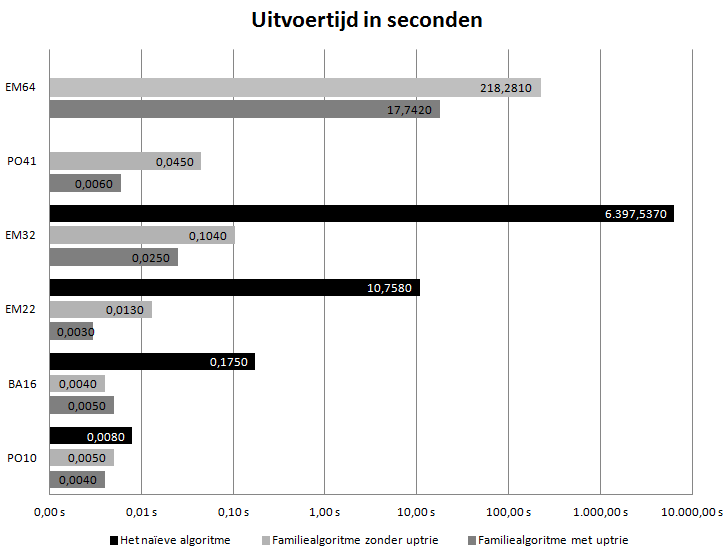Today I finally finished my thesis. It’s topic was to count the number of upper sets in partially ordered sets Which is quite a hard problem since it’s in the complexity class #P-Complete (that’s the class of counting the solutions to the decision problems in NP-complete). All and all I’m quite pleased with the result. Although the upper bound is still [latex]O(2^{n})[/latex], (can’t quite get under there without solving P=NP and winning a million dollars) I’ve manged to find a solution that has a best case of [latex]O(n)[/latex] both in time and memory complexity. With a particularly large data set the brute-force algorithm took over 2 hours to complete while my algorithm took 0.025 seconds. Now that’s what I’d call a speed gain (and yes it was a real life data set, no tricks here). You can see this for yourself in the graph at the bottom of this post the ‘naïeve algoritme’ is the brute force approach, the ‘Familiealgoritme zonder uptrie’ is the first version of my algorithm, the ‘Familiealgoritme met uptrie’ is the final version of my algorithm. It uses a trie like data structure to speed up searching and uses a lot less memory. Note that the graph has a logarithmic scale.

Unfortunately for most readers my thesis is in Dutch, but I’ve translated the abstract to English:

Counting the number of upper sets in partially ordered sets gives us a unique number that can be used to compare sets. This number is like the fingerprint of a set. Until now there isn't, as to my knowledge, an efficient algorithm to calculate this number. This meant that the number had to be calculated either by hand or by using a brute force approach. Using a brute force approach leads quickly to problems, even for trivially small data sets since this means that you have to generate 2^n subsets and check each of these subsets on upwards closure. When calculating by hand you can use symmetry but this menial process can take a lot of time and is error prone. In this thesis I present an algorithm that can calculate exact, and usually fast, the number of upper sets in a partially ordered set.

You can download my thesis here: Upper sets in partially ordered sets (Bsc thesis Roy Triesscheijn) as I’ve said before the text is in Dutch, but the proofs and attached code should be readable enough. If you’ve got any questions feel free to ask below!